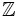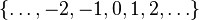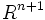# Ring of rational integers

See all particular commutative unital rings

## Definition

The ring$\mathbb{Z}$, called the ring of rational integers or sometimes simply the ring of integers, is the ring whose elements are the rational integers, with the usual addition and multiplication. Explicitly, the underlying set is$\{ \dots, -2, -1, 0, 1, 2, \dots \}$ and the addition and multiplication are the usual ones.

The adjective rational is used in the name in circumstances where there may be potential confusion with the ring of integers in a number field.

This ring is the initial object in the category of commutative unital rings.

## Ring properties

Property Meaning Satisfied? Explanation
integral domain zero is not a product of nonzero elements Yes
Euclidean domain admits a Euclidean norm Yes See ring of rational integers is Euclidean with norm equal to absolute value (the standard choice of norm is the absolute value); see also ring of rational integers is Euclidean with norm equal to binary logarithm of absolute value
principal ideal domain (PID) every ideal is a principal ideal Yes Follows from being Euclidean and Euclidean implies PID
unique factorization domain every element has a unique factorization into irreducibles (same as primes) up to units Yes Follows from being a PID and PID implies UFD
Noetherian domain integral domain Yes Follows from being a PID
Bezout domain Yes Follows from being a PID
Dedekind domain Yes Follows from being a PID
interpolation domain Yes For any$n$, there exists a tuple of$n + 1$ elements such that evaluation at these defines a bijection between the polynomials of degree at most$n$ in the ring of integer-valued polynomials and$R^{n+1}$.